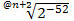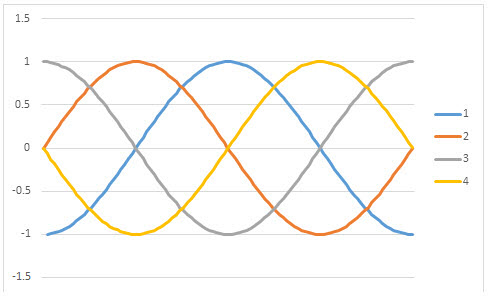# SQL Server FDERIV function

FDERIV
Updated: 15 April 2018

Use the scalar function FDERIV for function differentiation for orders n = 1 to 4 using finite difference approximations.

Syntax
SELECT [wct].[FDERIV] (
<@Func, nvarchar(max),>
,<@VarName, nvarchar(4000),>
,<@X, float,>
,<@N, int,>
,<@H, float,>
,<@Meth, nvarchar(4000),>)
Arguments
 Input Name Description @Func The function to be evaluated, as a string. The function must be in the form of a SELECT statement. @VarName The name of the variable @X The starting value for the evaluation @N Order of derivative, should only be between 1 and 8; for n = 0 function values will be returned @H Step size @Meth ‘C’, ‘B’, or ‘F’ for central finite difference, backward finite difference, or forward finite difference
Return Type
[float]
Remarks
• If @Func returns a NULL then NULL Is returned.
• If @Func is not a valid SELECT statement then NULL is returned.
• If no solution is found then NULL is returned.
• If @X is NULL then @X = 0.
• If @N is NULL then @N = 1.
• If @H is NULL then @H = 0.
• If @Meth is NULL then @Meth = 0.
• If @H <= 0 then @H =• Available in XLeratorDB / math 2008 only
Examples
Example #1

Calculate f(x) as sin x, d(sin x)/dx, and cos x

SELECT
SIN(PI()/4) as [sin x]
,wct.FDERIV(
'SELECT SIN(@x)'
,'@x'
,PI()/4
,1
,NULL
,'B'
) as [d(sin x)/dx]
,COS(PI()/4) as [cos x]

This produces the following result.

Example #2

Calculate the 1st through 4th derivatives of sin x from -PI to PI and past the results in a Excel PivotChart.

SELECT
n.n
,SeriesValue
,wct.FDERIV(
'SELECT SIN(@x)'
,'@x'
,SeriesValue
,n.n
,NULL
,'22'
)
FROM wct.SeriesFloat(-PI(),PI(),.02*PI(),NULL,'L')
CROSS APPLY (VALUES (1),(2),(3),(4))n(n)

This produces the following result.Copyright 2008-2021 Westclintech LLC         Privacy Policy        Terms of Service